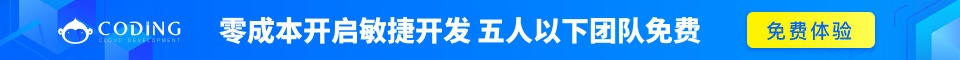# ASP.NET Razor C# 变量

## 变量

### 实例

// Using the var keyword:
var greeting = "Welcome to ziqiangxuetang.com";
var counter = 103;
var today = DateTime.Today;

// Using data types:
string greeting = "Welcome to ziqiangxuetang.com";
int counter = 103;
DateTime today = DateTime.Today;

## 数据类型

int整数（全数字）103, 12, 5168
float浮点数3.14, 3.4e38
decimal十进制数字（高精度）1037.196543
bool布尔值true, false
string字符串"Hello ziqiangxuetang.com", "John"

## 运算符

C# 语言支持多种运算符。下面列出了常用的运算符：

=给一个变量赋值。i=6
+
-
*
/

i=5+5
i=5-5
i=5*5
i=5/5
+=
-=

i += 1
i -= 1
==相等。如果值相等则返回 true。if (i==10)
!=不等。如果值不等则返回 true。if (i!=10)
<
>
<=
>=

if (i<10)
if (i>10)
if (i<=10)
if (i>=10)
+连接字符串（一系列互相关联的事物）。"w3" + "schools"
.点号。分隔对象和方法。DateTime.Hour
()圆括号。将值进行分组。(i+5)
[]中括号。访问数组或者集合的值。name
&&
||

## 转换数据类型

AsInt()
IsInt()

{myInt=myString.AsInt();}
AsFloat()
IsFloat()

{myFloat=myString.AsFloat();}
AsDecimal()
IsDecimal()

{myDec=myString.AsDecimal();}
AsDateTime()
IsDateTime()

myDate=myString.AsDateTime();
AsBool()
IsBool()

myBool=myString.AsBool();
ToString()转换任何数据类型为字符串。myInt=1234;
myString=myInt.ToString();